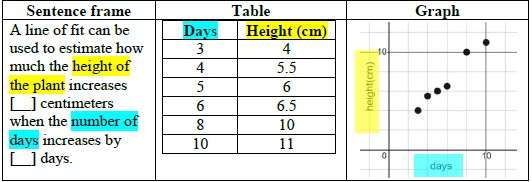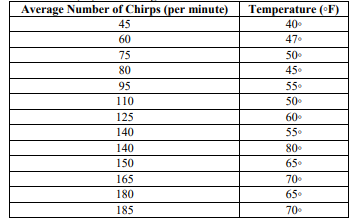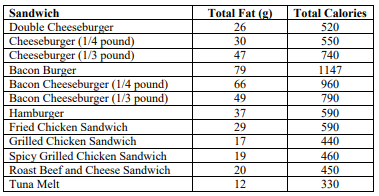# MA.912.DP.2.4Export Print
Fit a linear function to bivariate numerical data that suggests a linear association and interpret the slope and y-intercept of the model. Use the model to solve real-world problems in terms of the context of the data.

### Clarifications

Clarification 1: Instruction includes fitting a linear function both informally and formally with the use of technology.

Clarification 2: Problems include making a prediction or extrapolation, inside and outside the range of the data, based on the equation of the line of fit.

General Information
Subject Area: Mathematics (B.E.S.T.)
Strand: Data Analysis and Probability
Status: State Board Approved

## Benchmark Instructional Guide

### Terms from the K-12 Glossary

• Numerical Data
• Line of Fit
• Scatter Plot

### Vertical Alignment

Previous Benchmarks

Next Benchmarks

### Purpose and Instructional Strategies

In grade 8, students first worked with scatter plots and lines of fit. In Algebra I, students relate the slope and $y$-intercept of a line of fit to association in bivariate numerical data and interpret these features in real-world contexts. In later courses, students use the correlation coefficient to measure how well a line fits the data in a scatter plot, and they also work with scatter plots that suggest quadratic and exponential models.
• This is an extension of MA.912.DP.1.1, where students are working with numerical bivariate data (scatter plots and line graphs). It is good to review with students that a scatter plot is a display of numerical data sets between two variables.
• They are good for showing a relationship or association between two variables.
• They can reveal trends, shape of trend or strength of relationship trend.
• They are useful for highlighting outliers and understanding the distribution of data.
• One variable could be the progression of time, like in a line graph.
• In this benchmark, students are fitting a linear function to numerical bivariate data, interpreting the slope and $y$-intercept based on the context and using that linear function to make predictions about values that correspond to parts of the graph that lie beyond or within the scatter plot.
• Instruction includes the use of technology for students to understand the difference between a line of fit and a line of best fit. Additionally, instruction of this benchmark should be combined with MA.912.DP.2.6 and MA.912.DP.2.5, as these are extensions of this benchmark.
• During instruction is important to distinguish the difference between a “line of fit” and the “line of best fit.”
• A “line of fit” is used when students are visually investigating numerical bivariate data that appears to have a linear relationship and can sketch a line (using a writing instrument and straightedge) that appears to “fit” the data. Using this “line of fit” students can estimate its slope and $y$-intercept and use that information to interpret the context of the data.
• The “line of best fit” (also referred to as a “trend line”) is used when the data is further analyzed using linear regression calculations (the process of minimizing the squared distances from the individual data values to the line), often done with the assistance of technology.

### Common Misconceptions or Errors

• Students may not know how to sketch a line of fit.
• For example, they may always go through the first and last points of data.
• Students may be confuse the two variables when interpreting the data as related to the context.
• Students may not know the difference between interpolation (predictions within a data set) and extrapolation (predictions beyond a data set).

### Strategies to Support Tiered Instruction

• Teacher provides sketched lines of fit and has students identify the one that best models the data.
• Teacher provides a sentence frame for interpreting the data in the context of the problem using two different colored highlighters to highlight the same variable in the sentence frame and table or graph.
• Example:• Teacher models creating a scatter plot on a piece of graph paper, then has students place a piece of spaghetti on the scatter plot to model the line of best fit. Students could also use a coordinate plane peg board to plot each point creating a scatter plot and then use a rubber band to model the line of best fit.
• Instruction includes vocabulary development by co-creating a graphic organizer for interpolation and extrapolation.

Instructional Task 1 (MTR.4.1, MTR.5.1, MTR.6.1
• Crickets are one of nature’s more interesting insects, partly because of their musical ability. In England, the chirping or singing of a cricket was once considered to be a sign of good luck. Crickets will not chirp if the temperature is below 40 degrees Fahrenheit (°F) or above 100 degrees Fahrenheit (°F). A table is given with some data collected.• Part A. Create a line of fit based on the data. Compare your line of fit with a partner.
• Part B. What is the estimated slope and $y$-intercept of the line?
• Part C. What does the slope mean in terms of the context?
• Part D. What does the $y$-intercept mean in terms of the context?
• Part E. Using technology, determine the line of best fit. Compare this to the line of fit determine from Part A. What is the difference?
• Part F. Based on this line, predict the temperature to be if you recorded 250 chirps per minute?
• Part G. Based on this line, estimate the number of chirps per minute at exactly 50°F.

### Instructional Items

Instructional Item 1
• Below is data from a variety of fast food chains.• Part A. Create a scatter plot based on the data above and estimate an equation for a line of fit.
• Part B. What do the slope and $y$-intercept tell us about the relationship of total fat and total calories in these fast food items?
• Part C. If a fast food item has 10 grams of fat, estimate the total calories of that item.

*The strategies, tasks and items included in the B1G-M are examples and should not be considered comprehensive.

## Related Courses

This benchmark is part of these courses.
1200310: Algebra 1 (Specifically in versions: 2014 - 2015, 2015 - 2022, 2022 and beyond (current))
1200320: Algebra 1 Honors (Specifically in versions: 2014 - 2015, 2015 - 2022, 2022 and beyond (current))
1200370: Algebra 1-A (Specifically in versions: 2014 - 2015, 2015 - 2022, 2022 and beyond (current))
1200400: Foundational Skills in Mathematics 9-12 (Specifically in versions: 2014 - 2015, 2015 - 2022, 2022 and beyond (current))
1210300: Probability and Statistics Honors (Specifically in versions: 2014 - 2015, 2015 - 2019, 2019 - 2022, 2022 and beyond (current))
7912070: Access Mathematics for Liberal Arts (Specifically in versions: 2014 - 2015, 2015 - 2018, 2018 - 2019, 2019 - 2022, 2022 - 2023, 2023 and beyond (current))
7912080: Access Algebra 1A (Specifically in versions: 2014 - 2015, 2015 - 2018, 2018 - 2019, 2019 - 2022, 2022 and beyond (current))
1200315: Algebra 1 for Credit Recovery (Specifically in versions: 2014 - 2015, 2015 - 2022, 2022 and beyond (current))
1200375: Algebra 1-A for Credit Recovery (Specifically in versions: 2014 - 2015, 2015 - 2022, 2022 and beyond (current))
7912075: Access Algebra 1 (Specifically in versions: 2014 - 2015, 2015 - 2018, 2018 - 2019, 2019 - 2022, 2022 and beyond (current))
1210305: Mathematics for College Statistics (Specifically in versions: 2022 and beyond (current))
1207350: Mathematics for College Liberal Arts (Specifically in versions: 2022 and beyond (current))
1200388: Mathematics for Data and Financial Literacy Honors (Specifically in versions: 2022 and beyond (current))
1200384: Mathematics for Data and Financial Literacy (Specifically in versions: 2022 and beyond (current))
7912120: Access Mathematics for Data and Financial Literacy (Specifically in versions: 2022 - 2023, 2023 and beyond (current))

## Related Access Points

Alternate version of this benchmark for students with significant cognitive disabilities.
MA.912.DP.2.AP.4: Fit a linear function to bivariate numerical data that suggest a linear association and interpret the slope and y-intercept of the model.

## Related Resources

Vetted resources educators can use to teach the concepts and skills in this benchmark.

## Formative Assessments

Tuition:

Students are asked to use a linear model to make a prediction about the value of one of the variables.

Type: Formative Assessment

Foot Length:

Students are asked to interpret the line of best fit, slope, and y-intercept of a linear model.

Type: Formative Assessment

Swimming Predictions:

Students are asked to use a linear model to make and interpret predictions in the context of the data.

Type: Formative Assessment

House Prices:

Students are asked to informally fit a line to model the relationship between two quantitative variables in a scatterplot, write the equation of the line, and use it to make a prediction.

Type: Formative Assessment

Slope for Human Foot Length Model:

Students are asked to interpret the meaning of the slope of the graph of a linear model.

Type: Formative Assessment

Slope for Life Expectancy:

Students are asked to interpret the meaning of the slope of the graph of a linear model.

Type: Formative Assessment

Intercept for Life Expectancy:

Students are asked to interpret the intercept of a linear model of life expectancy data.

Type: Formative Assessment

Bungee Cord Model:

Students are asked to interpret the meaning of the constant term in a linear model.

Type: Formative Assessment

## Lesson Plans

Students will explore voter turnout data for three gubernatorial elections before and after the passage of the 19th amendment. They will fit linear functions to the data and compute predicted values for raw and percentage of voter turnout. Students will draw some conclusions concerning the relationship between eligible voters and voter turnout, including possible causes behind the fluctuation in voter participation in this integrated lesson

Type: Lesson Plan

What happens to available energy as it moves through an ecosystem?:

This activity is a lab exercise where students look at the passing of water in cups and compare it to the loss of available energy as it moves through an ecosystem. Students will collect data, calculate efficiency, graph the data and respond to reflection questions to connect the data to what happens in an ecosystem. The end of the activity includes a connection to the 10% rule where only 10% of energy from one trophic level is available at the next level.

Type: Lesson Plan

Mass Mole Relationships: A Statistical Approach To Accuracy and Precision:

The lesson is a laboratory-based activity involving measurement, accuracy and precision, stoichiometry and a basic statistical analysis of data using a scatter plot, linear equation, and linear regression (line of best fit). The lesson includes teacher-led discussions with student participation and laboratory-based group activities.

Type: Lesson Plan

How Fast Can You Go:

Students will apply skills (making a scatter plot, finding Line of Best Fit, finding an equation and predicting the y-value of a point on the line given its x-coordinate) to a fuel efficiency problem and then consider other factors such as color, style, and horsepower when designing a new coupe vehicle.

Type: Lesson Plan

Scatter plots, spaghetti, and predicting the future:

Students will construct a scatter plot from given data. They will identify the correlation, sketch an approximate line of fit, and determine an equation for the line of fit. They will explain the meaning of the slope and y-intercept in the context of the data and use the line of fit to interpolate and extrapolate values.

Type: Lesson Plan

## Perspectives Video: Experts

Oceanography & Math:

A discussion describing ocean currents studied by a physical oceanographer and how math is involved.

Type: Perspectives Video: Expert

Assessment of Past and Present Rates of Sea Level Change:

In this video, Brad Rosenheim describes how Louisiana sediment cores are used to estimate sea level changes over the last 10,000 years. Video funded by NSF grant #: OCE-1502753.

Type: Perspectives Video: Expert

Analyzing Antarctic Ice Sheet Movement to Understand Sea Level Changes:

In this video, Eugene Domack explains how past Antarctic ice sheet movement rates allow us to understand sea level changes. Video funded by NSF grant #: OCE-1502753.

Type: Perspectives Video: Expert

## Perspectives Video: Professional/Enthusiasts

Determining Strengths of Shark Models based on Scatterplots and Regression:

Chip Cotton, fishery biologist, discusses his use of mathematical regression modeling and how well the data fits his models based on  his deep sea shark research.

Type: Perspectives Video: Professional/Enthusiast

Slope and Deep Sea Sharks:

Shark researcher, Chip Cotton, discusses the use of regression lines, slope, and determining the strength of the models he uses in his research.

Type: Perspectives Video: Professional/Enthusiast

Linear Regression for Analysis of Sea Anemone Data:

Will Ryan describes how linear regression models contribute towards his research on sea anemones.

Type: Perspectives Video: Professional/Enthusiast

Mathematically Modeling Eddy Shedding :

COAPS oceanographer Dmitry Dukhovskoy describes the process used to mathematically model eddy shedding in the Gulf of Mexico.

Type: Perspectives Video: Professional/Enthusiast

Analyzing Wildlife Data Trends with Regression :

Dr. Bill McShea from the Smithsonian Institution discusses how regression analysis helps in his research.

This video was created in collaboration with the Okaloosa County SCIENCE Partnership, including the Smithsonian Institution and Harvard University.

Type: Perspectives Video: Professional/Enthusiast

## STEM Lessons - Model Eliciting Activity

How Fast Can You Go:

Students will apply skills (making a scatter plot, finding Line of Best Fit, finding an equation and predicting the y-value of a point on the line given its x-coordinate) to a fuel efficiency problem and then consider other factors such as color, style, and horsepower when designing a new coupe vehicle.

## MFAS Formative Assessments

Bungee Cord Model:

Students are asked to interpret the meaning of the constant term in a linear model.

Foot Length:

Students are asked to interpret the line of best fit, slope, and y-intercept of a linear model.

House Prices:

Students are asked to informally fit a line to model the relationship between two quantitative variables in a scatterplot, write the equation of the line, and use it to make a prediction.

Intercept for Life Expectancy:

Students are asked to interpret the intercept of a linear model of life expectancy data.

Slope for Human Foot Length Model:

Students are asked to interpret the meaning of the slope of the graph of a linear model.

Slope for Life Expectancy:

Students are asked to interpret the meaning of the slope of the graph of a linear model.

Swimming Predictions:

Students are asked to use a linear model to make and interpret predictions in the context of the data.

Tuition:

Students are asked to use a linear model to make a prediction about the value of one of the variables.

## Student Resources

Vetted resources students can use to learn the concepts and skills in this benchmark.

## Parent Resources

Vetted resources caregivers can use to help students learn the concepts and skills in this benchmark.